In 1669, Jungius disproved Galileo's claim that the curve of a chai...
Tables of common logarithms were used until the invention of comput...
Here's an animation of Leibniz's recipe ![](https://i.imgur.com/...
Read lebniz paper citation  for clarity
A way to determine y is to write the expression \frac{dy}{dx}=\sqr... It's also important to note that John Nappier's work on logarithms ...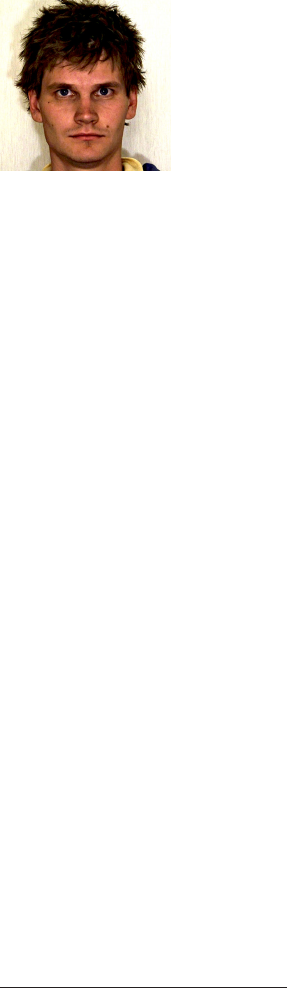How to Find the Logarithm of Any Number Using Nothing But a Piece of String Viktor Bl ˚ asj ¨ o Viktor Bl ˚ asj ¨ o (v.n.e.blasjo@uu.nl) studied at Stockholm University and the London School of Economics and received his Ph.D. in the history of mathematics at Utrecht University, with a dissertation on the representation of curves in the 17th century. In his spare time, Bl ˚ asj ¨ o enjoys photography; a favorite motif is the geometry of industrial landscapes. The shape of a freely hanging chain suspended from two points is called the catenary, from the Latin word for chain. In principle, any piece of string would do, but one speaks of a chain since a chain with ﬁne links embodies in beautifully concrete form the ideal physical assumptions that the string is nonstretchable and that its elements have complete ﬂexibility independent of each other. In modern terms, the catenary can be expressed by the equation y = (e x + e x )/2. As we shall see, Leibniz did not state this formula explicitly, but he understood well the relation it expresses, calling it a “wonderful and elegant harmony of the curve of the chain with logarithms” [6, p. 436]. (English translations of  and  are given in .) Indeed, he continued, the close link between the catenary and the exponen- tial function means that logarithms can be determined by simple measurements on an actual catenary. “This may be helpful since during long journeys one may lose one’s table of logarithms ...In case of need the catenary can then serve in its place” [7, p. 152]. Leibniz’s recipe for determining logarithms in this way is delightfully simple and can easily be carried out in practice using, for example, a cheap necklace pinned to a cardboard box with sewing needles. Leibniz’s recipe Refer to Figure 1 and the following description. (a) Suspend a chain from two horizontally aligned nails. Draw the horizontal through the endpoints, and the vertical axis through the lowest point. (b) Put a third nail through the lowest point and extend one half of the catenary horizontally. (c) Connect the endpoint to the midpoint of the drawn horizontal, and bisect the line segment. Drop the perpendicular through this point, draw the horizontal axis through the point where the perpendicular intersects the vertical axis, and take the distance from the origin of the coordinate system to the lowest point of the catenary to be the unit length. We will show below that the catenary now has the equation y = (e x + e x )/2 in this coordinate system. http://dx.doi.org/10.4169/college.math.j.47.2.95 MSC: 01A45 VOL. 47, NO. 2, MARCH 2016 THE COLLEGE MATHEMATICS JOURNAL 95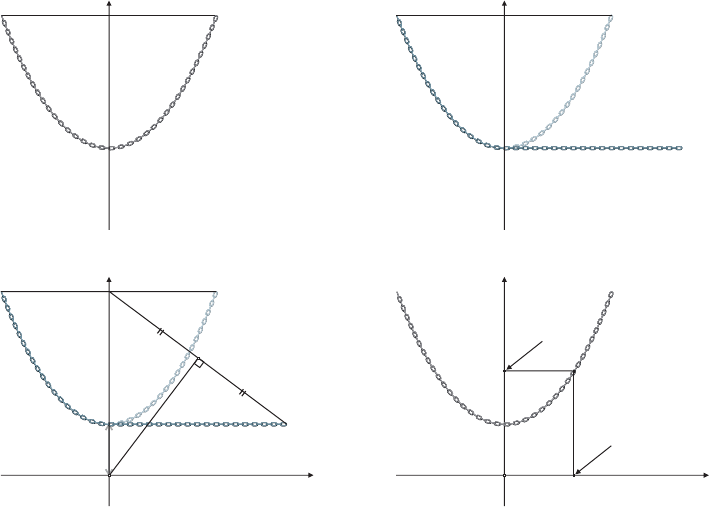(a) (b) (c) (d) (Y +1/Y )/2 log(Y ) Figure 1. Leibniz’s recipe for determining logarithms from the catenary. (d) To ﬁnd log(Y ),ﬁnd(Y + 1/Y )/2onthey-axis and measure the corresponding x-value (on the catenary returned to its original form). This assumes that Y > 1. To ﬁnd logarithms of negative values, use the fact that log(1/Y ) =−log( Y ).If you seek the logarithm of a very large value, then you may end up too high on the y-axis; in such cases you can either try hanging the endpoints closer together or using logarithm laws to express the desired logarithm in terms of those of lower values. The last step in this construction is given in Leibniz’s catenary papers [5, 7, 8] where, however, the preceding steps are implicit at best; Leibniz later spelled these steps out in [11, No. 199]. The validity of this construction may be conﬁrmed as follows. Figure 2 shows the forces acting on a segment of a catenary starting from its lowest point: the tension forces at the endpoints, which act tangentially, and the gravitational force, which is proportional to the arc s from T 0 to T . Since the catenary is in equilibrium, it is evident that the horizontal and vertical components of T balance with T 0 and the weight as, respectively, so T x =−T 0 and T y =−as. But since T acts in the direc- tion of the tangent, we also know T y /T x = dy/dx. Thus we obtain dy/dx = as/T 0 . On the left half of the catenary, where s is negative, we get instead T y = as and T y /T x = dy/dx, which gives the same result. For convenience we choose the units of force and mass so that a/T 0 = 1, which gives dy/dx = s as the differential equa- tion for the catenary. Squaring both sides of this equation and using the Pythagorean identity (dx) 2 + (dy) 2 = (ds) 2 to eliminate dx leads to (dy) 2 = s 2 (ds 2 dy 2 ) or (1 + s 2 )(dy) 2 = s 2 (ds) 2 and, by separating the variables and taking square roots, 96 © THE MATHEMATICAL ASSOCIATION OF AMERICA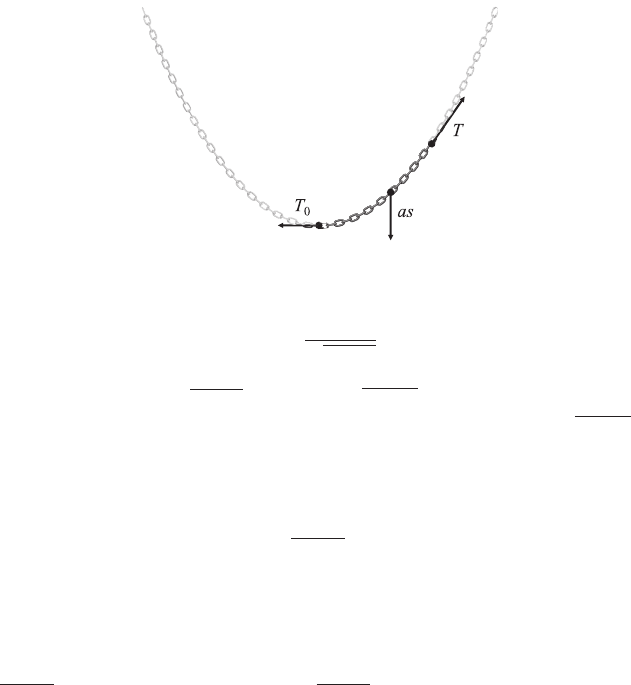Figure 2. The forces acting on a segment of a catenary. dy = sds 1 + s 2 , which integrates to y = 1 + s 2 . Thus s = y 2 1, which we can substitute into the original differential equation for the catenary to obtain dy/dx = y 2 1. It is now straightforward to check that y = (e x + e x )/2 is the solution to this differential equation that passes through (0, 1). It remains to verify that the coordinate system assumed in this solution is the same as that deﬁned by the construction of Figure 1. The key to Leibniz’s veriﬁcation turns out to be the intermediate step y = 1 + s 2 above. To see this, consider Figure 3, which is Figure 1(c) with additional notation. We know from above that the catenary FA L is given by y = (e x + e x )/2 in a certain coordinate system whose origin O is at a vertical distance OA = 1 below the lowest point of the catenary. Consider the particular y-value OH = y and the associated arc AL = s, then construct the horizontal segment AM with the same length s. It follows by the Pythagorean theorem that OM = 1 + s 2 . But above we saw that y = 1 + s 2 , which means in terms of this ﬁgure that OH = OM. Thus OHM is an isosceles triangle and so the perpendicular bisector of its base HM passes through the vertex O. This shows that the construction of Figure 1 does indeed give a way of recovering the coordinate system associated with the solution y = (e x + e x )/2, as we needed to show. From here it is a simple matter of algebra to check the ﬁnal step of Figure 1. In a 17th-centur y context Finding logarithms from a catenary may seem like an oddball application of mathe- matics today, but to Leibniz it was a very serious matter—not because he thought this method so useful in practice, but because it pertained to the very question of what it means to solve a mathematical problem. Today we are used to thinking of a formula such as y = (e x + e x )/2 as the answer to the question of the shape of the catenary, but this would have been considered a na ¨ ıve view in the 17th century. Leibniz and his contemporaries discovered this relation between the catenary and the exponential function in the 1690s, but they never wrote this equation in any form, even though they understood perfectly well the relation it expresses. Nor was this for lack of familiarity with exponential expressions, at least in Leibniz’s case, as he had earlier used such expressions to describe curves with considerable facility [11, No. 6]. Why, indeed, should one express the solution as a formula? What kind of solution to the catenary problem is y = (e x + e x )/2, anyway? The 17th-century philosopher VOL. 47, NO. 2, MARCH 2016 THE COLLEGE MATHEMATICS JOURNAL 97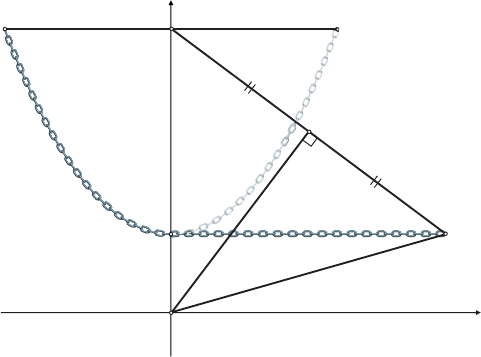O A H LF B M Figure 3. Figure used by Leibniz [11, No. 199] to justify the construction shown in Figure 1. Thomas Hobbes once quipped that the pages of the increasingly algebraical mathemat- ics of the day looked “as if a hen had been scraping there” [4, p. 330] and what indeed is an expression such as y = (e x + e x )/2 but some chicken-scratches on a piece of paper? It accomplishes nothing unless e x is known already, i.e., unless e x is more basic than the catenary itself. But is it? The fact that it is a simple formula of course proves nothing; we could just as well make up a symbolic notation for the catenary and then express the exponential function in terms of it. And, however one thinks of the graph of e x , it can hardly be easier to draw than hanging a chain from two nails. So why not reverse the matter and let the catenary be the basic function and e x the application? Modern tastes may have it that pure mathematics is primary and its applications to physics secondary, but what is the justiﬁcation for this hierarchy? Certainly none that would be very convincing to a 17th-century mind. The 17th-century point of view also had the authority of tradition on its side. Euclid’s Elements had been the embodiment of the mathematical method for two mil- lennia and one of its most conspicuous aspects is its insistence on constructions. Euclid never proves anything about a geometrical conﬁguration that he has not ﬁrst shown how to construct by ruler and compass. These constructions are what gave meaning to mathematics and deﬁned its ontology. This paradigm remained as strong as ever in the 17th century. When Descartes introduced analytic geometry in his G ´ eom ´ etrie of 1637, nothing was further from his mind than a scheme to replace the construction-based conception of mathematics by one centered on formulas. On the contrary, his starting point was a new curve-tracing method, which he presented as a generalization of the ruler and compass of classical geometry, and he accepted algebraic curves only once he had established that they could be generated in this manner . It is in this context that we must understand Leibniz’s construction: He sees the catenary not as an applied problem to be reduced to mathematical formulas, but as a fundamental construction device analogous to the ruler and the compass of Euclidean geometry. (See Figure 4 for two of his original ﬁgures.) Extending the constructional toolbox with new curve-tracing devices along these lines was a major research program in the late 17th century. Beside the catenary, other physical curves were also called upon for this purpose, such as the elastica  and the tractrix . 98 © THE MATHEMATICAL ASSOCIATION OF AMERICA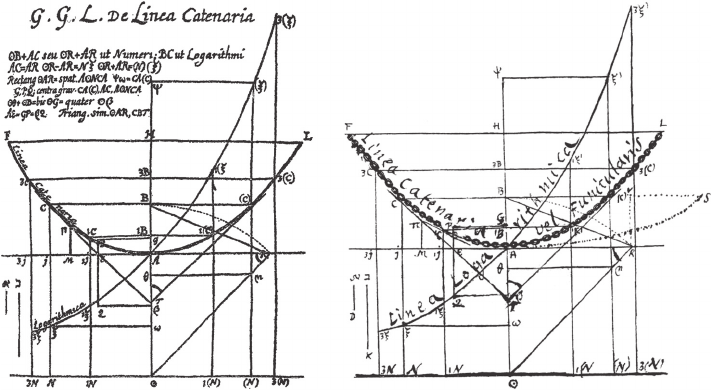(a) (b) Figure 4. Leibniz’s ﬁgures for the catenary, showing its relation to the exponential function. (From and, respectively.) Thus 17th-century mathematicians had reason to reject the “chicken-scratch math- ematics” that we take for granted today. They published not formulas but the con- crete, constructional meaning that underlies them. If you want mathematics to be about something, then this is the only way that makes sense. It is prima facie absurd to deﬁne mathematics as a game of formulas and at the same time to assume na ¨ ıvely a direct correspondence between its abstraction and the real world, such as y = (e x + e x )/2 with the catenary. It makes more sense to turn the tables: to deﬁne the abstract in terms of the concrete, the construct in terms of the construction, the exponential function in terms of the catenary. It was against this philosophical backdrop that Leibniz pub- lished his recipe for determining logarithms using the catenary. We see, therefore, that it was by no means a one-off quirk, rather it was a natural part of a concerted effort to safeguard meaning in mathematics. Summary. We present Leibniz’s 1691 recipe for determining logarithms using the catenary and discuss why this odd-looking application in fact made good sense in its historical context. References 1. V. Bl ˚ asj ¨ o, The rectiﬁcation of quadratures as a central foundational problem for the early Leibnizian calculus, Historia Math. 39 (2012) 405–431, http://dx.doi.org/10.1016/j.hm.2012.07.001. 2. H. J. M. Bos, Tractional motion and the legitimation of transcendental curves, Centaurus 31 (1988) 9–62, http://dx.doi.org/10.1111/j.1600-0498.1988.tb00714.x. 3. ———, Redeﬁning Geometrical Exactness: Descartes’ Transformation of the Early Modern Concept of Construction. Springer, New York, 2001. 4. T. Hobbes, The English works of Thomas Hobbes of Malmesbury. Vol. 7. Longman, Brown, Green, and Longmans, London, 1845. 5. G. W. Leibniz, De linea in quam ﬂexile se pondere proprio curvat, ejusque usu insigni ad inveniendas quot- cunque medias proportionales & logarithmos, Acta Eruditorum 10 (1691) 277–281. 6. ———, De solutionibus problematis catenarii vel funicularis in Actis Junii A. 1691, aliisque a Dn. I. B. propositis, Acta Eruditorum 10 (1691) 434–439. VOL. 47, NO. 2, MARCH 2016 THE COLLEGE MATHEMATICS JOURNAL 997. ———, De la chainette, ou solution d’un probl  eme fameux propos ´ e par Galilei, pour servir d’essai d’un nouvelle analise des inﬁnis, avec son usage pour les logarithmes, & une application  alavancementdela navigation, J. Sc¸avans (Mar. 1692) 147–153. 8. ———, Solutio illustris problematis a Galilaeo primum propositi de ﬁgura chordae aut catenae ex duobus extremis pendentis, pro specimine nouae analyseos circa inﬁnitum, Giornale de’ Letterati (Apr. 1692) 128–132. 9. ———, ¨ Uber die Analysis des Unendlichen. Ed., trans. G. Kowalewski. Engelmann, Leipzig, 1908. 10. ———, Two papers on the catenary curve and logarithmic curve, trans. P. Beaudry, Fidelio Mag. 10 no. 1 (Spring 2001) 54–61, http://www.schillerinstitute.org/fid_97-01/011_catenary.html. 11. , S ¨ amtliche Schriften und Briefe. Reihe III: Mathematischer, naturwissenschaftlicher und technischer Briefwechsel. Band 5: 1691–1693. Leibniz-Archiv, Hannover, 2003, http://www.leibniz-edition.de. Coming in Mathematics Magazine When are Finite Projective Planes Magic? by David A Nash and Jonathan Needleman Squigonometry, Hyperellipses, and Supereggs by Rob Poodiack Spacewalks and Amusement Rides: Illustrations of Geometric Phase by Jeffrey K. Lawson and Matthew Rave Bounds for the Representations of Integers by Positive Quadratic Forms by Kenneth Stuart Williams Building the Biggest Box: Three-Factor Polynomials and a Diophantine Equation by David Cruz-Uribe and Gregory Convertito Intransitive Dice by Brian Conrey, James Gabbard, Katie Grant, Andrew Liu, and Kent E. Morrison 100 © THE MATHEMATICAL ASSOCIATION OF AMERICA Read lebniz paper citation  for clarity In 1669, Jungius disproved Galileo's claim that the curve of a chain hanging under gravity would be a parabola (Red). The real shape is a catenary (Black). ![](https://i.imgur.com/2BFmTXI.gif) A way to determine y is to write the expression\frac{dy}{dx}=\sqrt{y^2-1}$in the form: $$y^2-\left(\frac{dy}{dx}\right)^2 =1$$ which is basically the same as the following identity involving the hyperbolic functions: $$cosh^2(x) - sinh^2(x) = 1$$ in which$y=cosh(x) = \frac{e^x-e^{-x}}{2}$and$\frac{dy}{dx}=sinh(x)= \frac{e^x+e^{-x}}{2}\$ It's also important to note that John Nappier's work on logarithms is from 1618. $$\lim_{n \to \infty} (1+1/n)^n$$ The discovery of the constant itself is credited to Jacob Bernoulli in 1683 and it was only in 1731 that Leonhard Euler introduced the letter "e" as the base for natural logarithms in a letter to Christian Goldbach. The name is _John Napier_ (https://en.m.wikipedia.org/wiki/John_Napier) 1550-1617. His major work (on logarithms among other things) was published in 1614. Tables of common logarithms were used until the invention of computers and electronic calculators to do rapid multiplications, divisions, and exponentiations, including the extraction of nth roots. These tables were particularly useful in long sea trips, in which daily determination of latitude and longitude were needed. In theory, this method could be used as a backup to build a logarithmic table on board of ship if needed. ![](https://upload.wikimedia.org/wikipedia/commons/thumb/7/71/Logarithmorum_Chilias_Prima_page_0-67.jpg/800px-Logarithmorum_Chilias_Prima_page_0-67.jpg) Here's an animation of Leibniz's recipe ![](https://i.imgur.com/stQ7vg5.gif)